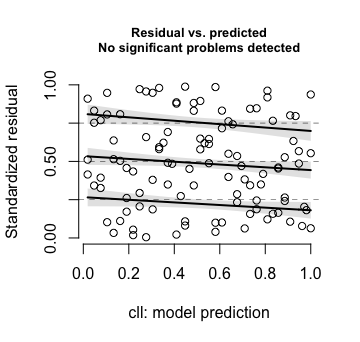# Introduction

Quantal responses are counts of all-or-none responses, out of a total $$n$$. A dose-response relationship quantifies the response as a function of dose, or more generally as a function of an exposure.

Data are from a broad class of experiments where responses are insect deaths out of some total number exposed. Exposure may be time in coolstorage, or dose of a fumigant, or a concentration-time measure of exposure to a fumigant, or an intensity-time measure of exposure to radiation. See Follett and Neven (2006) for commentary on the regulatory and scientific background. We will use Hawaian fruitfly data that has been supplied by Dr Peter Follett to demonstrate the use of functions in the qra package, where the exposure is time in coolstorage,

The following code sets up the data.

HawCon <- qra::HawCon
## Change name "CommonName" to "CN", for more compact output.
CCnum <- match("CommonName", names(HawCon))
names(HawCon)[CCnum] <- "CN"
## trtGp will identify species & lifestage combination
## trtGpRep will identify species, lifestage, and rep
## cTime is centered version of TrtTime
## scTime is centered and scaled version of TrtTime,
## needed to get some mixed model fits to converge
HawCon <- within(HawCon, {
trtGp <- factor(paste0(CN,LifestageTrt, sep=":"))
trtGpRep <- paste0(CN,LifestageTrt,":",RepNumber)
scTime <- scale(TrtTime)
obs <- factor(1:nrow(HawCon))
})

# 1 Data setup and choice of model

For the data that will be considered here, the exposure measure is time in coolstorage, and the response is mortality of insect pests.

## 1.1 Graphical Display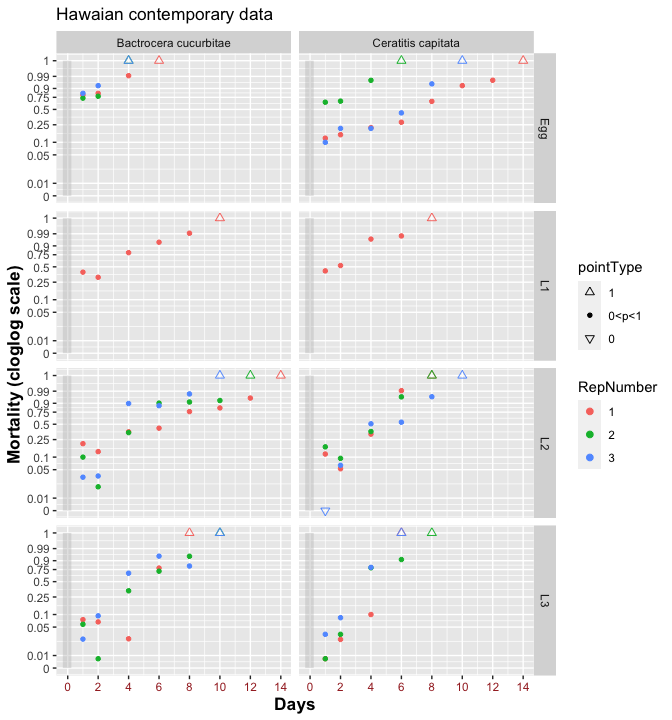Figure 1.1: Graphs are designed to give an indication of the pattern, when mortalities are shown on a complementary log-log scale, of mortality response with days in coolstorage.

Figure 1.1 is designed to give a broad indication of the pattern of response, on a complementary log-log link scale. Responses appear acceptably linear, at least after the first one or two observations. There are clear systematic differences between replicates, indicative of a strong between replicate component of variation.

## 1.3 Modeling the error distribution

With data such as here, it is to be expected that the response will show strong extra-binomial variation. This happens because the response varies from insect to insect, and/or because insects do not respond independently. The glmmTMB package (Brooks et al. 2017) implements the betabinomial error family, with the option to model the scale parameter, and hence the multiplier for the binomial variance, as a function of explanatory variable(s). In the terminology used for R’s glm() function, and that will be used in the sequel, the multiplier for the binomial variance is referred to as the dispersion factor. For the data considered here, this dispersion factor is high in the middle of the range of data, reducing at the extremes.

An alternative that will be investigated in a later section is the use of binomial errors, with observation level random effects used to account for differences at the level of individual observations. For the present data, this automatically achieves much the same effect as betabinomial errors, without the need for specific attention to the modeling of a dispersion factor. The model fit appears, however to be less satisfactory than the use of betabinomial errors with a dispersion factor adjustment.

Other error models that are described in the literature, and that operate at the level of individual observations, are discussed in the vignette vignette("countDists", package = "qra").

## 1.4 Choices required for mixed model fits

Fits will require a choice of link functions, modeling of the fixed effects, and modeling of the error distribution. Because there are just three replicates per lifestage, it is necessary to base estimates on a model that brings together components of variance information across lifestages. This inevitably leads to estimates that will sometimes smooth out effects that are specific to an individual lifestage.

The subsection that follows will explore the use of a betabinomial error model, as implemented in the glmmTMB package. The parameterization is that described in Morris (1997), with parameters $$\mu$$ and $$\phi$$. Here $$\mbox{E}[x] = n \mu$$, and $\mbox{var}[x] = n \mu (1-\mu) \dfrac{\phi+n}{\phi+1}$ Setting $$\phi = \frac{1-\rho}{\rho}$$, where $$\rho$$ is the intra-class correlation, this equals $n \mu(1-\mu)(1+(n-1)\rho)$

### Quasibinomial errors

The lme4::glmer() function offers the option of a quasibinomial error, as for stats::glm(). It does not offer an equivalent to the glmmTMB dispformula.

Specification of a quasibinomial error has the consequence that the model is fitted as for a binomial distribution, with the the binomial variance $$n \pi (1- \pi)$$ then multiplied by a constant factor $$\Phi$$ that is usually estimated using the Pearson chi-squared statistic. Compare this with the betabinomial, where the multiplier is $$\Phi = 1+(n-1)\rho$$, i.e., it increases with $$n$$. This is an important difference.

## 1.6 Fitted lines, vs fitted normal spline curves

Figure 1.2 shows fitted lines, and fitted degree 2 normal spline curves, in Panel A for a complementary log-log (cloglog) link, and in Panel B for a logit link.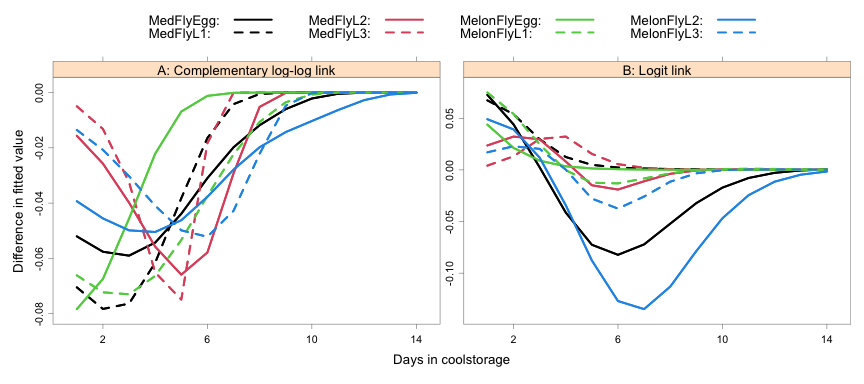Figure 1.2: Differences are shown, between fitted degree 2 normal spline curves and fittes lines. Panel A is for the models that use a complementary log-log (cloglog) link, while Panel B is for a logit link.

## 1.7 Diagnostic checks

Randomized quantile residuals, with the plots that are available in the DHARMa provide helpful diagnostic check. For any residual, the corresponding quantile residual is the proportion of residuals expected, under model assumptions, to be less than or equal to it. If the distributional assumptions are satisfied, the quantile residuals should have a distribution that differs only by statistical error from a uniform distribution.

The function DHARMa::simulateResiduals() provides a convenient means to simulate enough sets of residuals (by default, 250) to give a good sense, for each individual observation, of the distribution. These then provide a reference distribution for calculation of quantile residuals. Residuals may be calculated allowing only for the fixed effects (the default), or conditional on one or more levels of random effects. If the model is correct, residuals should be uniformly distributed irrespective of the conditioning. See ?DHARMa::simulateResiduals for details.

• For the data as a whole, the distribution of residuals can be checked by plotting the quantile residuals against the corresponding quantiles.
• Departures from assumptions show a pattern of difference from the line y=x that is different from that for normal distribution quantile-quantile plots.
• A second check plots quantile residuals against quantiles of predicted values. Quantile regression is then used to fit curves at 25%, 50%, and 75% quantiles of the quantile residuals. If the model is correctly specified, these should all be, to within statistical error, horizontal lines.
• Plots against other explanatory variables provide added checks.

Do such deviations from assumptions as are present matter?
A useful device is to simulate new ‘observations’ from the model, and check whether there is a difference of substance in the fitted values and parameter estimates.

Figure 1.3 shows the diagnostic plots for the linear model with a complementary log-log link.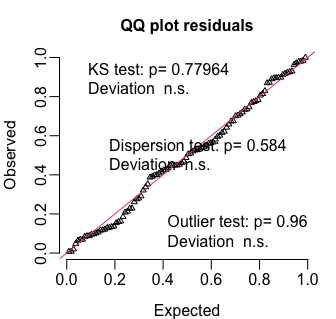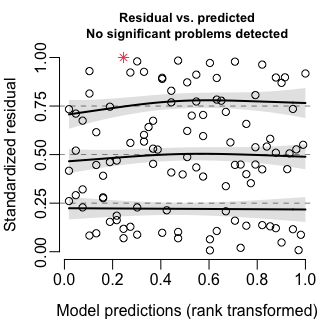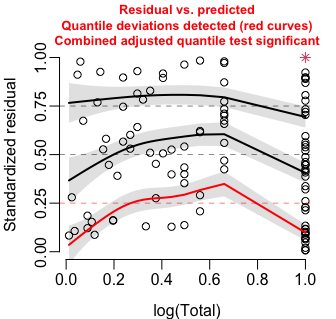Figure 1.3: Panel A shows the quantile-quantile plot, for the linear model with a complementary log-log link. Panel B plots estimated quantiles against mortality, while Panel C plots estimated quantiles against total number, on a logarithmic scale.

The quantile-quantile (Q-Q) plot looks fine, The quantile residuals from the data appear, if anything, closer to uniformly distributed than any of the simulated sets of residuals. In Panels B and C, the quartiles of the data are appear satisfactorily close to the relevant quartiles.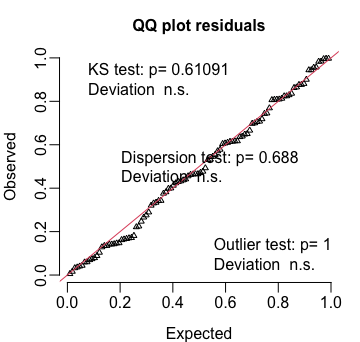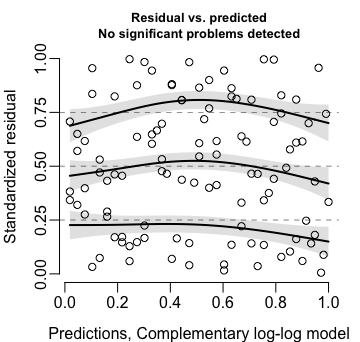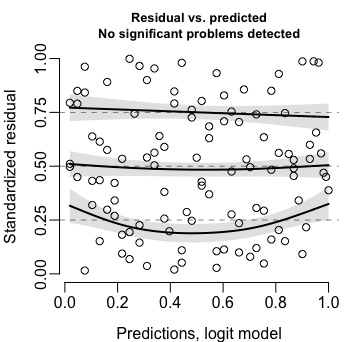Figure 1.4: Diagnostic plots, for the model with a logit link.

Now compare (Figure 1.4) scaled residuals between treatment groups.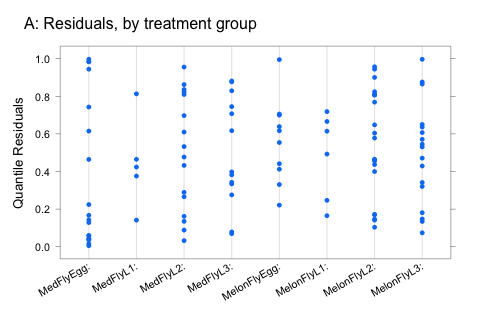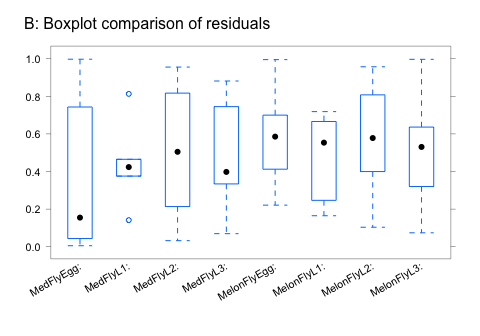Figure 1.5: Quantile residuals, by treatment group, for the betabinomial model

The numbers for MelonEgg, MedL1, and MelonL1 are too small to give useful boxplot displays. Except for MedEgg, where points are concentrated in the tails, the scatter of points in Panel A appears reasonably comparable between treatment groups.

QQ plots look good for all the models. In the sequel, they will be left out.

### Uniform quantile-quantile plots — an example.

We will generate data from a highly overdispersed binomial type distribution, then examine the uniform quantile-quantile plot given by DHARMa::plotResiduals(). An easy way to generate overdispersed binomial type data is to start with binomial data, then multiply both “successes” and “failures” by a number that is substantially greater than one.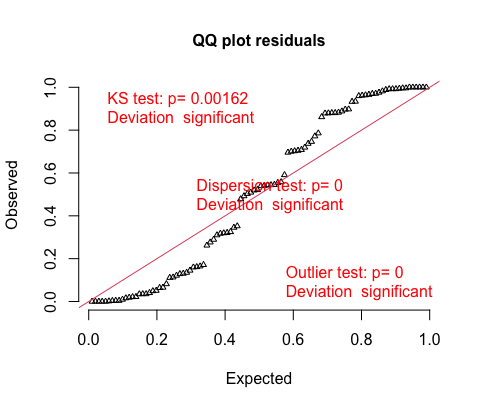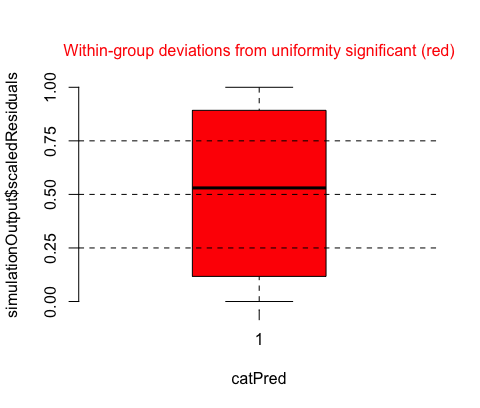Figure 1.6: Diagnostics for model fitted to strongly overdispersed binomial type data. Notice that the overdispersion results in an S-shaped distribution of the residuals around the line $$y=x$$. The boxplot is, in this case, uninformative.

### AIC-based model comparisons

Comparisons should be for models with the same outcome variable, and with the same data.2

In addition to the models described so far, we will include also models, to be discussed below, that assume binomial errors, with observational level random effects added. The model with the lowest AIC is the preferred model.

The following shows AIC values

    bb.cll bb2s.cll bb.logit bb2s.logit biObs.cll biObs.logit
df   27.00       29    27.00      29.00     20.00       20.00
AIC 710.01       NA   721.68     720.02    719.33      721.29

There is a strong preference for a complementary log-log link, with the linear fit slightly better than the degree 2 normal spline fit, arguing for the use of the fitted lines for calculation of LT99s and confidence intervals.

## 1.8 Estimates of $$\rho$$, and of the dispersion factor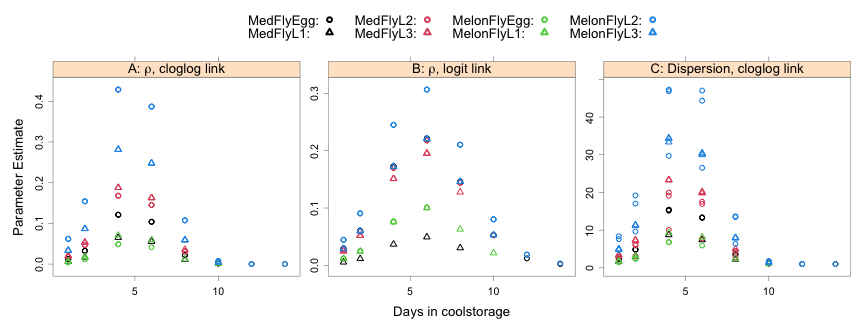Figure 1.7: Panels A and B show intra-class correlation estimates for, respectively, a complementary log-log link and a logit link. Both models assume a betabinomial error. Panel C shows, for the complementary log-log model, the dispersion factors that result.

Figures 1.7A and B (for a logit link) plot the estimates of $$\rho$$, based on modeling the logarithm of the scale parameter as a degree 2 natural spline function of scTime that is added to a straight line response that is different for each different treatment group. Use of a logarithmic link (the default) has the consequence that effects that are additive on the link scale become multipliers when unlogged.

Figure 1.7C shows, for the complementary log-log model, the dispersion factors that result — high at midrange times and mortalities, reducing to close to a constant 1.0 at either extreme. Use of a normal spline basis helps ensures that the value for $$\rho$$, and hence the dispersion factor, extrapolates to a close to constant value at both extremes.

A dispersion factor that is close to 1.0 at the upper extreme of the data provides a limited justification for assuming a binomial distribution, for purposes of calculating the sample size needed to demonstrate, at a 95% confidence level, a mandated survival rate of, e.g., no more than one in perhaps 100,000 insects.

# 2 Binomial errors, plus observation level random effects

The suggestion here is that for the replicates within each species/lifestage combination, the response in any one observation is close to binomial. Added to this is random variation between observations. By comparison with fitting a betabinomial or a quasibinomial error, the effect is to reduce the variance of the observed mortalities at low and high mortality points, relative to variance at midrange values.

The following fits the two models: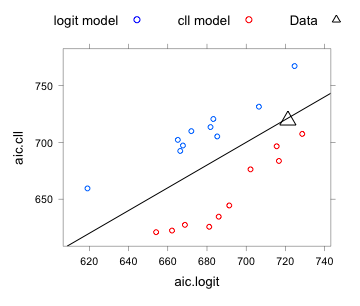Figure 2.1: AICs are compared between models with binomial family errors and observation level random effects. The two sets of points make the comparison for, respectively, data that have been simulated from a model with logit link and a model with complementary log-log link. The large triangle makes the comparison for the models fitted to the ‘HawCon’ data.

Notice that the random effects term (scTime|trtGpRep) has been changed to (1|trtGpRep). This avoids convergence failure messages.

The AIC statistic shows a slight preference for the complementary log-log model. The comparison with data that have been simulated from the respective fitted distributions indicates that the distinction is far from clear.

The attempt to repeat a similar comparison with models that assume a betabinomial error failed. Most simulationa generated error messages.

Figure 2.2 compares the diagnostic plots, between use of a complementary log-log link, and use of a logit link.